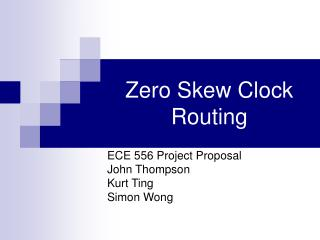# Zero Skew Clock Routing - PowerPoint PPT PresentationDownload PresentationZero Skew Clock Routing

Zero Skew Clock Routing
Download Presentation## Zero Skew Clock Routing

- - - - - - - - - - - - - - - - - - - - - - - - - - - E N D - - - - - - - - - - - - - - - - - - - - - - - - - - -
##### Presentation Transcript

1. Zero Skew Clock Routing ECE 556 Project Proposal John Thompson Kurt Ting Simon Wong

2. Overview • Problem definition • Nearest neighbor • Zero-skew tree construction • Algorithm • Benchmarks

3. Problem Definition • Given a set S of sink locations, and given a connection topology G, construct a zero skew clock tree T(S) with topology G and having minimum cost…

4. Nearest Neighbor • Use Delaunay Triangulation to find the pair of points with shortest separating distance. • These are the points that will be connected next…

5. Delaunay Triangulation • A Delaunay triangulation of a vertex set is a triangulation of the vertex set with the property that no vertex in the vertex set falls in the interior of the circumcircle (circle that passes through all three vertices) of any triangle in the triangulation.

6. Original vertex set Delaunay Triangulation Delaunay Triangulation

7. Delaunay Triagulation • Dual of Voronoi Diagram

8. Voronoi Diagram • N is the set of all sites S. • The Voronoi polygon vor(S) of a site SN is defined to be the region consisting of the points in Rd whose nearest neighbor in N is S. • Voronoi Diagram is the set of all vor(S). Vor(S)

9. Construction of Vonoroi Diagram • Partition N into subsets N1, N2 of equal sizes by median x-coordinates

10. Construction of Vonoroi Diagram • Construct Vor(N1) and Vor(N2) recursively

11. Construction of Vonoroi Diagram • Construct Vor(N1) and Vor(N2) recursively

12. Construction of Vonoroi Diagram • Construct dividing chain

13. Construction of Vonoroi Diagram • Discard all edges of Vor(N2) that lie to the left of dividing line, and the same for Vor(N1) • The result is Vor(N) is the Voronoi Diagram of the entire set

14. Approximation of Manhattan Segments • Use n points on a Manhattan Segment to represent it in Delaunay Triagulation

15. Zero-Skew Tree Construction • Use a clustering-based optimization algorithm • Algorithm steps • Find center • Embedding • Local Embedding

16. CL Find Center • Step 1: • K := S • Step 2: • Stop once the root node has been reached. Otherwise, construct the nearest-neighbor graph G(K,E) on K, and sort edges in E by their weights in non-decreasing order. • Step 3: • Take the smallest weight edge (v1, v2) from E, and delete the edge from E. Calculate segment for v from v1, v2. Delete v1, v2 from K, add v to K. If the capacitance of a subtree becomes too large, insert a buffer…

17. CL Find Center Pick K pairs of Near Neighbor for Manhattan Segment Calculation

18. Calculating Manhattan Segments • Three cases • From two points • From a point and a segment • From two segments • The general case is from two segments

19. Manhattan Segment Construction from Two Perpendicular Manhattan Segments

20. Merging Segments Segments after merging Manhattan Segments after Find Center

21. Simple Buffer Insertion Heuristic

22. Embedding • Step 1: • Determine the center vc on the segment for vc by selecting the nearest point to the root vr. Route from vr to vc. • Step 2: • Local embedding on vc…

23. Embedding VR VC Manhattan Segments after Find Center Determine Center Vc From Vr

24. Local Embedding • Step 1: • If v has no child, return… • Step 2: • Let v1 and v2 be children of v. Then determine the point vi on the segment for vi so as to satisfy the zero-skew merge equations. Route from v to vi… • Step 3: • Perform local embedding on v1 and v2…

25. Embedding VR VR VC Local Embedding for VC & remaining segments Final Routing Tree

26. Benchmarks • UCLA MCNC clock benchmark 1.0 suite • Originally from Jackson’s (et al.) “Clock routing for high performance ICs” paper… • UCLA IBM clock benchmark 1.0 suite • Originally R. S. Tsay’s benchmarks used in his “Exact zero skew” paper… • UCLA ISCAS clock benchmark 1.0

27. References • Masato Edahiro; “A Clustering-Based Optimization Algorithm in Zero-Skew Routings”; 1993 • Ting-Hai Chao, Yu-Chin Hsu, and Jan-Ming Ho; “Zero Skew Clock Net Routing”; 1992 • Ting-Hai Chao, Yu-Chin Hsu, Jan-Ming Ho, and Kenneth D. Boese; “Zero Skew Clock Routing with Minimum Wirelength”; 1992 • Yu Chen, Andrew B. Kahng, Gang Qu, and Alexander Zelikovsky; “The Associative-Skew Clock Routing Problem”; 1999